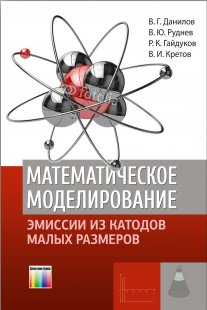• A
• A
• A
• ABC
• ABC
• ABC
• А
• А
• А
• А
• А
Regular version of the site

## Математическое моделирование эмиссии из катодов малых размеров

Данилов В. Г., Руднев В. Ю., Гайдуков Р. К., Кретов В. И.

A new mathematical model of heat transfer in silicon field emission pointed cathode of small dimensions is constructed which permits taking its partial melting into account. This mathematical model is based on the phase field system, i.e., on a contemporary generalization of Stefan-type problems. The approach used by the authors is not purely mathematical but is based on the understanding of the solution structure (construction and study of asymptotic solutions) and computer calculations. The book presents an algorithm for numerical solution of the equations of the obtained mathematical model including its parallel implementation. The results of numerical simulation conclude the book.

The book is intended for specialists in the field of heat transfer and field emission processes and can be useful for senior students and postgraduates.